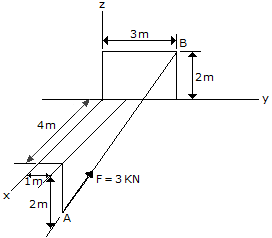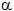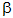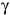# Engineering Mechanics - Force Vectors - Discussion

### Discussion :: Force Vectors - General Questions (Q.No.3)

3.Express force F as a Cartesian vector; then determine its direction angles.

 [A]. F = (-2i +j+2k) kN,= 48.2°,= 70.5°,= 48.2° [B]. F = (-2i +j+2k) kN,= 131.8°,= 70.5°,= 48.2° [C]. F = (-4i +j+4k) kN,= 48.2°,= 70.5°,= 48.2° [D]. F = (-4i +2j+4k) kN,= 131.8°,= 70.5°,= 48.2°

Explanation:

No answer description available for this question.

 Ramesh said: (Mar 12, 2012) I want the explaination. I can't understand.

 Benjamin said: (Apr 7, 2012) I am relearning a lot of maths and if there is anyone about that can write an method I would be very greatful, forgotten how this format works entirely.

 Avinash said: (Jun 19, 2012) Write the position vectors B and A. Then do A-B. Result is the vector direction.

 Wahid said: (Jun 6, 2014) A(4;1;-2) & B(0;3;2) then vect(AB) = 2(-2i+j+2k)and, 180-cos(a) = 2/AB then a = 131.8. cos(b) = 1/AB then b = 70.5. cos(c) = 2/AB then c = 48.2.

 .Shinde Mahesh said: (Jan 13, 2015) I thing its very lengthy process. Any one can give shortcuts for this problem?

 D Sanjog Roshan said: (Sep 9, 2017) A(4,1,-2) and B(0,3,2). Position vector, Rab=-4i+2j+4k Unit vector of Rab=-4/6i+2/6j+4/6k F=3(Rab)= option B. α=cos-1(-2/3)=131.8. β=cos-1(1/3)=70.5. γ=cos-1(2/3)=48.1.

 Hazim Khaleel said: (Jun 9, 2019) The right answer is D.